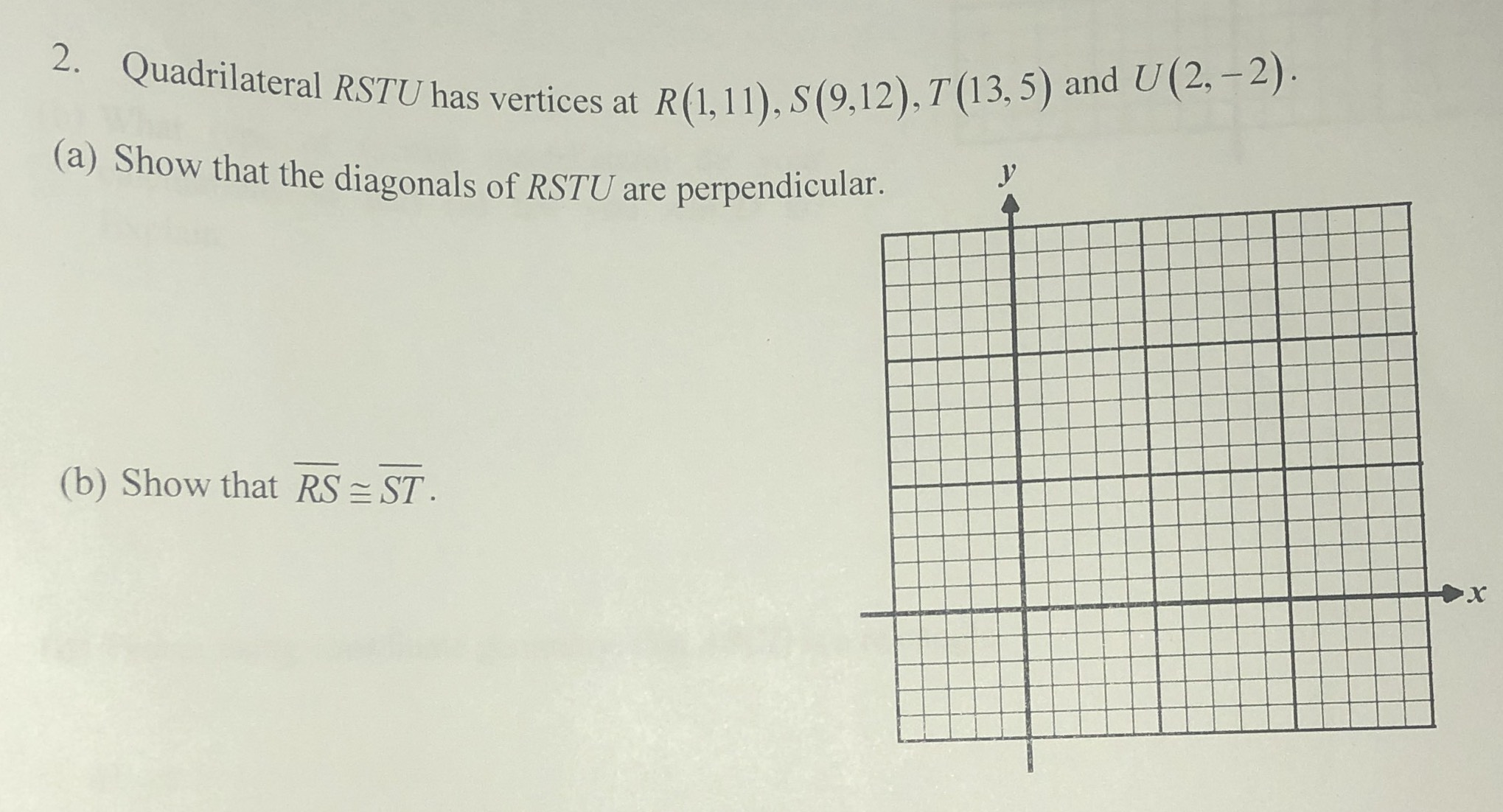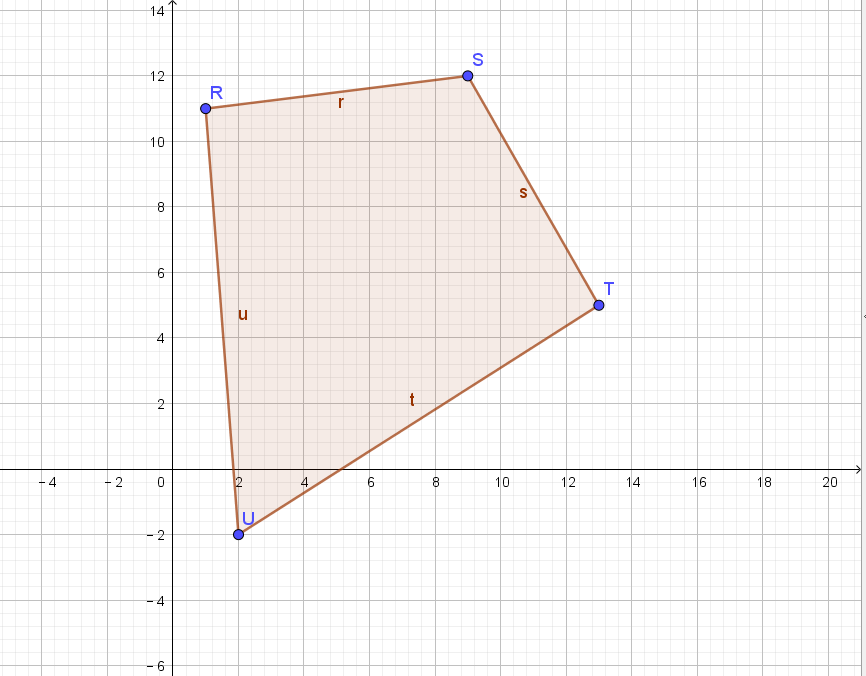### ¿Todavía tienes preguntas de matemáticas?

Pregunte a nuestros tutores expertos
Algebra
PreguntaQuadrilateral $$R S T U$$ has vertices at $$R ( 1,11 ) , S ( 9,12 ) , T ( 13,5 )$$ and $$U ( 2 , - 2 )$$ .

(a) Show that the diagonals of $$R S T U$$ are perpendicular.

(b) Show that $$\overline { R S } \cong \overline { S T }$$

(a)The polygon is drawn as follows:The slope of RT is $$k_{1}= - \frac{1}{2}$$,and the slope of SU is $$k_{2}= 2$$.

$$k_{1}k_{2}= - 1$$

(b)$$RS= \sqrt{8^{2}+ 1}= \sqrt{65}$$

$$ST= \sqrt{4^{2}+ (- 7)^{2}}= \sqrt{65}$$

so $$RS= ST$$

Solución
View full explanation on CameraMath App.